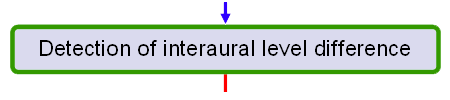# Detection of interaural level differenceThe interaural level difference is detected using a simple measurement. The mean sound pressure level (SPL) in each channel in each measurement window for each frequency band is calculated at the same time as the cross-correlation calculation. The SPL values for the left channel are then subtracted from the SPL values for the right channel. This is similar to the level-meter model proposed by Hartmann and Constan [Hartmann and Constan 2002]. The resulting data are then passed on to be combined with the measurement of the interaural time difference.